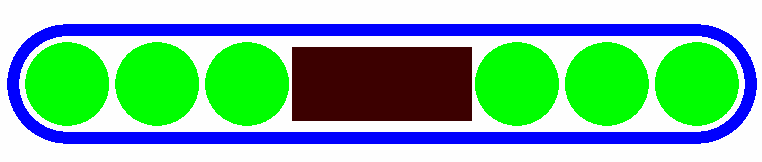# Best Industries, BestPd-103, 2335### Source Description:

Dimensions for the 2335 source are taken from studies by Meigooni et al 1, Peterson and Thomadsen 2, and Rivard  et al  3. The Best 2335 source consists of a cylindrical tungsten marker which is 1.20 mm long with a diameter assumed to be 0.50 mm. On either side of the marker are three 0.560 mm diameter spheres with centres located at ± 0.90 mm, ± 1.50 mm, and ± 2.10 mm from the middle of the seed (Note: The BrachyDose model used in the previous version of this database used 0.50 mm diameter spheres). The spheres are made of a polymer (composition by weight of 89.73% C, 7.85% H, 1.68% O and 0.740% N, and the density of the polymer is assumed to be 1.0 g/cm3). The polymer spheres are coated with 103Pd which is assumed to have negligible thickness. The polymer spheres and tungsten marker are encapsulated in a 0.080 mm thick Ti casing with an outer diameter of 0.80 mm. The end welds are assumed to be hemispherical shells with a thickness of 0.080 mm. The unoccupied space inside the seed is assumed to be air.The overall source length is 5.00 mm and the active length is 4.76 mm which is calculated according to the TG43U1 recomendation as described in ref. 4 (which differs from the value 4.55 mm used in TG43U1S15). The maximum possible displacement of a source sphere is 0.570 mm along the seed axis and up to 0.070 mm in the radial direction, however, we assume the six beads are always centred. The mean photon energy calculated on at the surface of the source is 20.54 keV with statistical uncertainty < 0.01%

### Dose-Rate Constant - Λ :

Dose-rate constants, Λ , are calculated by dividing the dose to water per history in a (0.1 mm)3 voxel centered on the reference position,        (1 cm,Π/2), in a 30x30x30 cm3 water phantom, by the air-kerma strength per history (scored in vacuo). As described in ref.  , dose-rate constants are provided for air-kerma strength calculated using voxels of 2.66x2.66x0.05 cm3 (WAFAC) and 0.1x0.1x0.05 cm3 (point) located 10 cm from the source. The larger voxel size averages the air kerma per history over a region covering roughly the same solid angle subtended by the primary collimator of the NIST WAFAC 7, 8  used for calibrating low-energy brachytherapy sources and is likely the most clinically relevant value. The small voxel serves to estimate the air kerma per history at a point on the transverse axis and includes a small 1/r2 correction (0.5%) 6MC uncertainties are only statistical uncertainties (k=1).

### Radial dose function - g(r):

The radial dose function, g(r), is calculated using both line and point source geometry functions and tabulated at 36 different radial distances ranging from 0.05 cm to 10 cm. Fit parameters for a modified polynomial expression are also provided 13. The mean residual deviation from the actual data for the best fit is < 0.15%.

Click image for higher res version### Anisotropy function - F(r,θ):

Anisotropy functions are calculated using the line source approximation and tabulated at radii of 0.1, 0.15, 0.25, 0.5, 0.75, 1, 2, 3, 4, 5, 7.5 and 10 cm and 32 unique polar angles with a minimum resolution of 5° . The anisotropy factor, φan (r), was calculated by integrating the solid angle weighted dose rate over 0° ≤ ϑ ≤ 90°.

### Tabulated data:

Tabulated data are available in .xlsx format: Excel